Question

# I. Write VHDL statement to perform the following operation using simple arithmetic and logic circuits: Y...

I. Write VHDL statement to perform the following operation using simple arithmetic and logic circuits:

Y = (3X*(8X + 6))/2 mod 28,

where X and Y are 16-bit unsigned numbers. Minimize the number of multipliers and dividers used in your circuit. You can ignore overflow. (8 points)

`library ieee;`
```use ieee.std_logic_1164.all;
```
```entity examp is
Port ( X : in  STD_LOGIC_VECTOR (15 downto 0);    -- 16-bit number
Y : out  STD_LOGIC_VECTOR (15 downto 0));    -- 16-bit result

end examp;

architecture Behavioural of examp is
```
```    signal X : STD_LOGIC_VECTOR (15 downto 0);
signal Y : STD_LOGIC_VECTOR (15 downto 0);```
```begin
Y<= (3 * X *(8 * X + 6)) / 2 mod 28;
end Behavioural;```

#### Earn Coins

Coins can be redeemed for fabulous gifts.

Similar Homework Help Questions
• ### FIRST ACTIVITY: (100/100) . SIMPLE 4-BIT ARITHMETIC LOGIC UNIT (ALU): This circuit selects between arithmetic (absolute...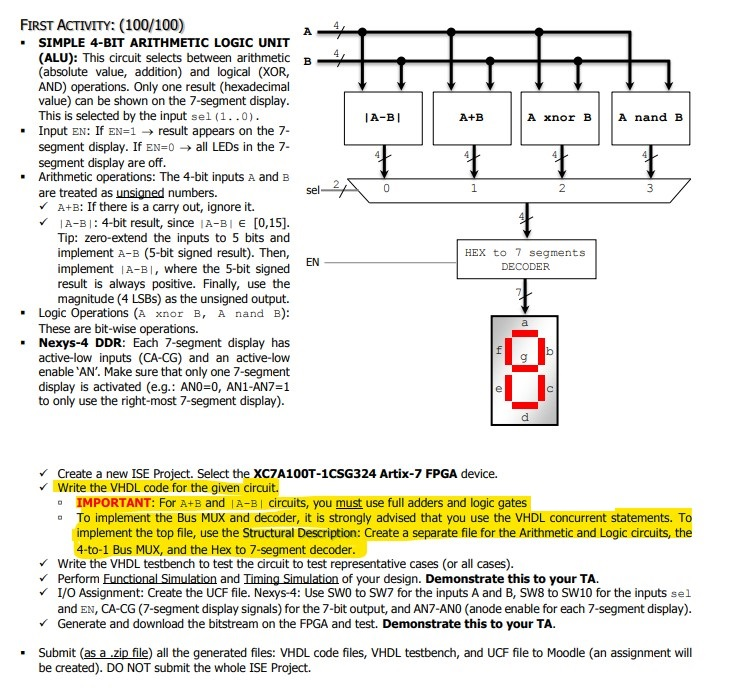FIRST ACTIVITY: (100/100) . SIMPLE 4-BIT ARITHMETIC LOGIC UNIT (ALU): This circuit selects between arithmetic (absolute value, addition) and logical (XOR, AND) operations. Only one result (hexadecimal value) can be shown on the 7-segment display This is selected by the input sel (1..0) B A-BI A+B A xnor B A nand B Input EN: If EN-1result appears on the 7 segment display. If EN=0 → all LEDs in the 7 segment display are off Arithmetic operations: The 4-bit inputs A...

• ### Using Structural Modeling in VHDL write the code for: An Arithmetic Logic Unit (ALU) shown in...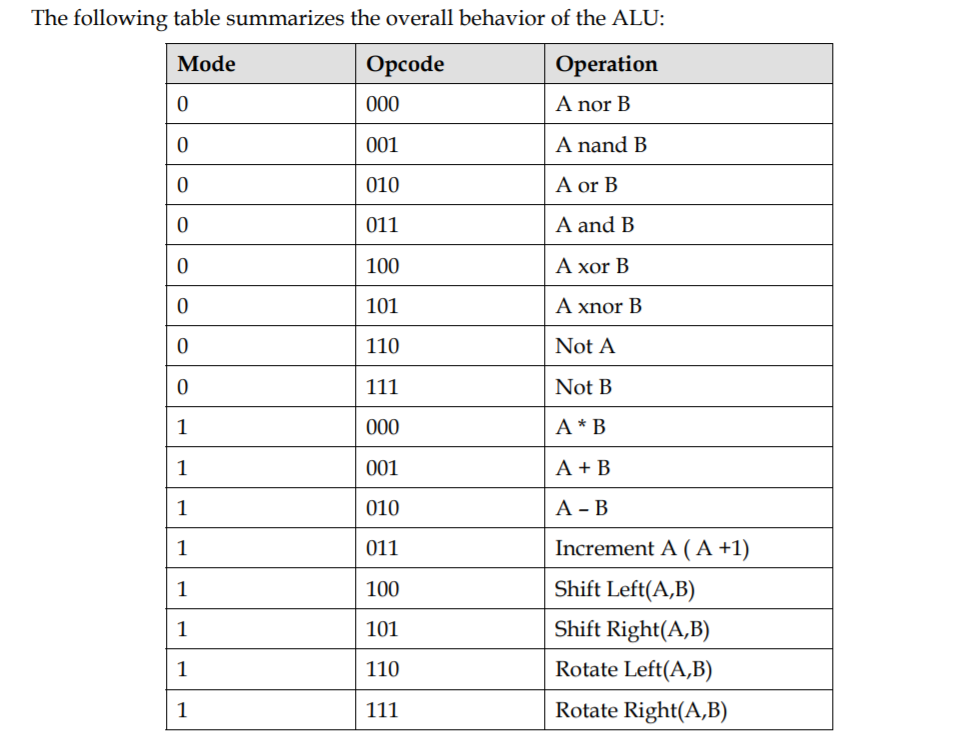Using Structural Modeling in VHDL write the code for: An Arithmetic Logic Unit (ALU) shown in the figure below. A (16-bit), B (16-bit), Opcode (3-bit), and Mode (1-bit) are the inputs; and ALUOut (16-bit) and Cout (1-bit) are the outputs of the design. A and B hold the values of the operands. Mode and Opcode together indicate the type of the operation performed by ALU. The ALU components ARE: -Arithmetic Unit that consists of one 16-bit adder, 16-bit subtractor, 16-bit...

• ### PROBLEM STATEMENT The mini-calculator will use a small ALU to perform arithmetic operations on two 4-bit values which are set using switches. The ALU operations described below are implemented with a...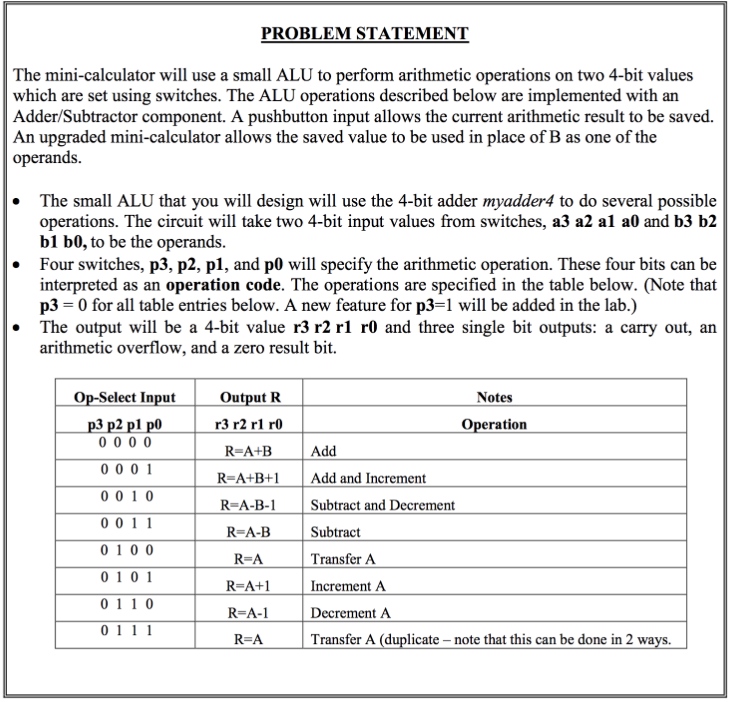PROBLEM STATEMENT The mini-calculator will use a small ALU to perform arithmetic operations on two 4-bit values which are set using switches. The ALU operations described below are implemented with an Adder/Subtractor component. A pushbutton input allows the current arithmetic result to be saved. An upgraded mini-calculator allows the saved value to be used in place of B as one of the operands. The small ALU that you will design will use the 4-bit adder myadder4 to do several possible...

• ### 26. The is a group of bits that tells the computer to perform a specific operation...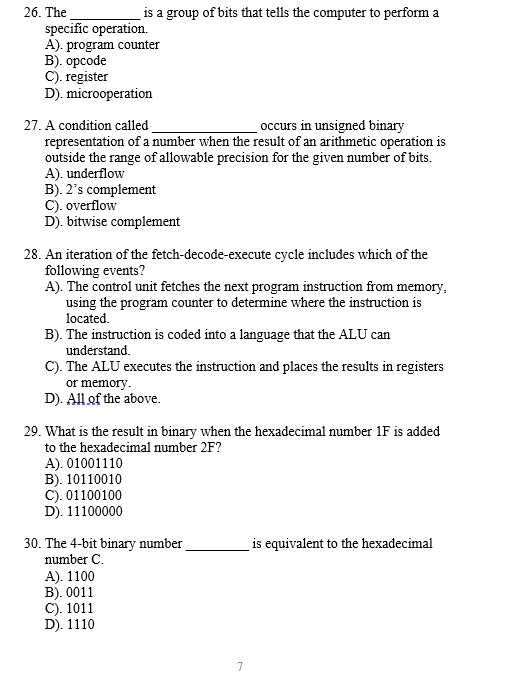26. The is a group of bits that tells the computer to perform a specific operation A). program counter B). Opcode C). register D). microoperation 27. A condition called occurs in unsigned binary representation of a number when the result of an arithmetic operation is outside the range of allowable precision for the given number of bits. A). underflow B). 2's complement C). overflow D) bitwise complement 28. An iteration of the fetch-decode-execute cycle includes which of the following events?...

• ### 1. (15 pts) Simplify the following Boolean functions using K-maps: a. F(x,y,z) = (1,4,5,6,7) b. F(x,...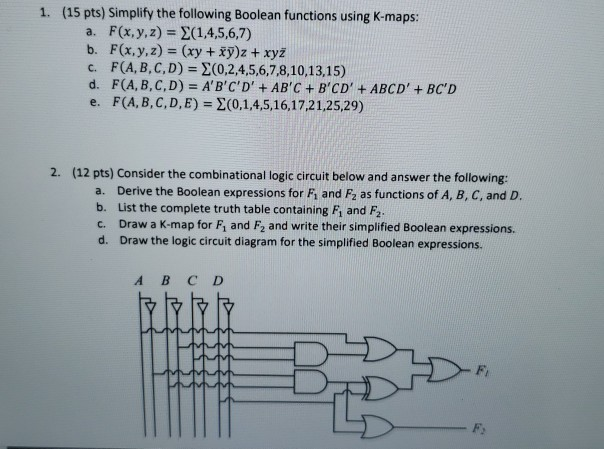1. (15 pts) Simplify the following Boolean functions using K-maps: a. F(x,y,z) = (1,4,5,6,7) b. F(x, y, z) = (xy + xyz + xyz c. F(A,B,C,D) = 20,2,4,5,6,7,8,10,13,15) d. F(A,B,C,D) = A'B'C'D' + AB'C + B'CD' + ABCD' + BC'D e. F(A,B,C,D,E) = (0,1,4,5,16,17,21,25,29) 2. (12 pts) Consider the combinational logic circuit below and answer the following: a. Derive the Boolean expressions for Fi and F2 as functions of A, B, C, and D. b. List the complete truth table...

• ### what is the largest prime number you will need to check to find all the prime numbers less than 100 Here is a site that I found searching Google with the key words, "<I>prime numbers</I>

what is the largest prime number you will need to check to find all the prime numbers less than 100Here is a site that I found searching Google with the key words, "prime numbers." http://mathforum.org/dr.math/faq/faq.prime.num.html I hope this helps. Thanks for asking.Finding Primes The Sieve of Eratosthenes Lets find the primes between 1 and 100. Write down the sequence of numbers from 1 to 100. Cross out the 1. Beginning with the 2, strike out every second number beyond the...